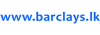## Computer Ram Prices in Sri Lanka

Showing 21 - 40 of 84 results for Computer Rams
Sort By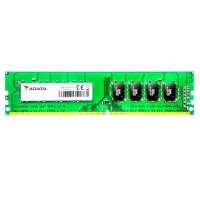Rs. 14,580   at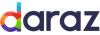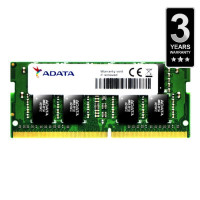Rs. 14,645   at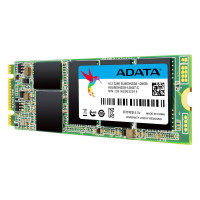Rs. 8,787   at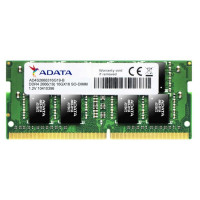Rs. 27,012   at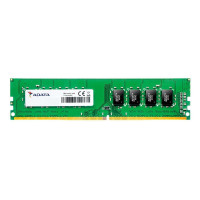Rs. 27,012   at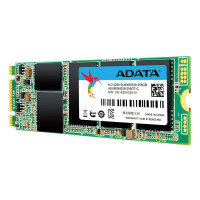Rs. 12,692   at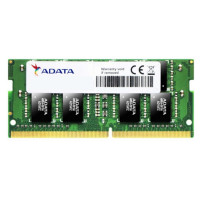Rs. 7,811   at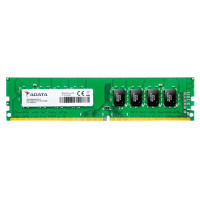Rs. 7,811   at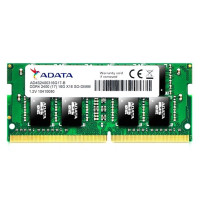Rs. 7,811   at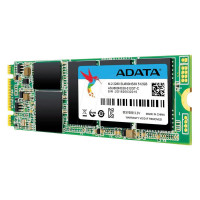Rs. 23,757   at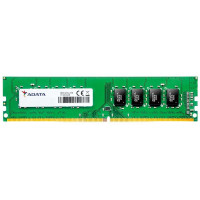Rs. 14,580   at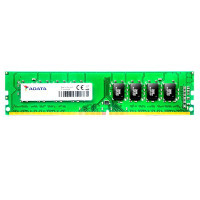Rs. 14,580   at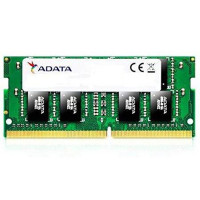Rs. 14,645   at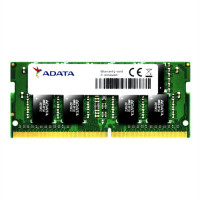Rs. 14,645   at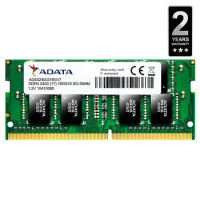Rs. 7,673   at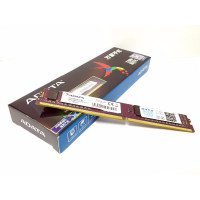Rs. 2,400   at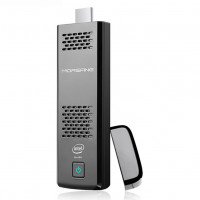Rs. 30,707   at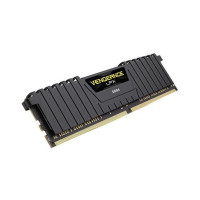Rs. 23,280   at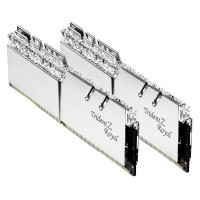Rs. 44,700   atRs. 35,500   at